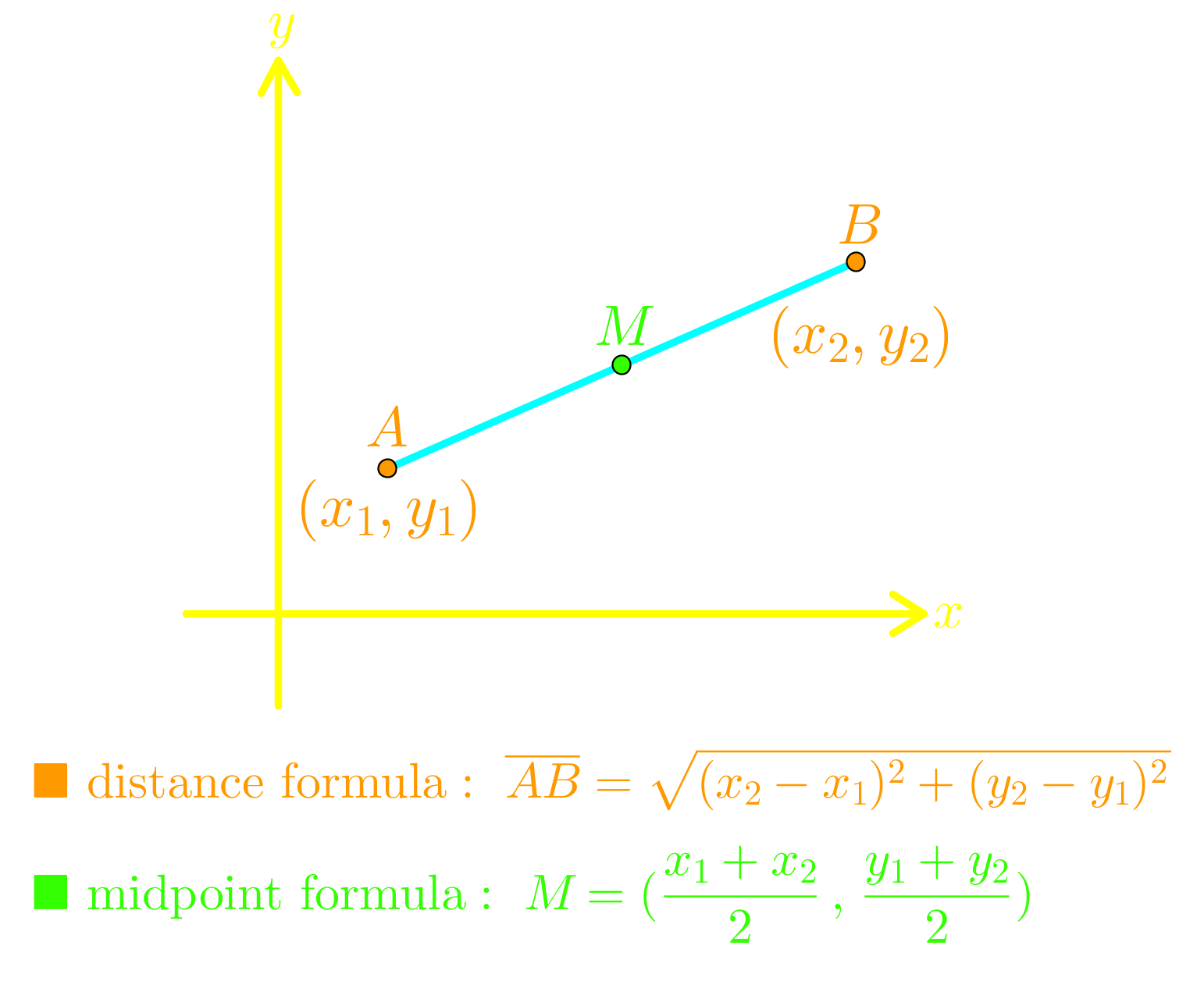# Conics - Circle

### Conics - Circle

#### LessonsThe conics form of a circle with center $\left( {h,\;k} \right)$ and radius $r$ is:
${\left( {\frac{{x - h}}{r}} \right)^2} + {\left( {\frac{{y - k}}{r}} \right)^2} = 1$
• 1.
graphing a circle
Sketch each circle and state the:
i) center
a)
${\left( {\frac{{x - 5}}{3}} \right)^2} + {\left( {\frac{{y + 4}}{3}} \right)^2} = 1$

b)
${x^2} + {y^2} = 25$

• 2.
converting a circle equation to conics form by "completing the square"
$4{x^2} + 4{y^2} + 24x - 8y + 15 = 0$
a)
Convert the equation to conics form.

b)
Sketch the graph and state the:
i) center

• 3.
finding the equation of a circle given its properties
Find the equation of a circle with:
a)
center $\left( { - 3,\;5} \right)$, radius = 7

b)
center $\left( {2,\;0} \right)$, passing through the point $\left( { - 1,\;4} \right)$

c)
diameter with endpoints $\left( { - 9,\;4} \right)$ and $\left( {15,\; - 6} \right)$

d)
center $\left( { - 2,\; - 1} \right)$, tangent to the line $3x + 4y = 15$